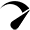# Solar Panel Size Calculator – Charge Your Battery In Desired Hours

Use our solar panel size calculator to find out what size solar panel you need to charge your battery in desired time.

Simply enter the battery specifications, including Ah, volts, and battery type. Also the charge controller type and desired charge time in peak sun hours into our calculator to get your result

Two columns
Vertical
Horizontal

## watts

{{ field.label }} {{ field.converted }} {{ field.extraView }}
{{ option.label }} {{ option.converted }}
{{ item.label }} {{ item.data.converted }}

Issued on: {{ \$store.getters.getIssuedOn }}
Payment method: {{ \$store.getters.getPaymentType }}
{{ item.label }}: {{ item.converted }}

### Calculator Assumptions

1. Battery charge efficiency rate: Lead-acid – 85%, AGM – 85%, Lithium (LiFePO4) – 99%
2. Charge controller efficiency: PWM – 80%; MPPT – 98% 
3. Solar Panels Efficiency during peak sun hours: 80%, this means that a 100 watt solar panel will produce 80 watts during peak sun hours. Click here to read more.
4. There are no devices drawing power from the battery during the charging process.

## How To Use Our Solar Panel Size Calculator?

1. Enter battery Capacity in amp-hours (Ah): For a 100ah battery, enter 100. If the battery capacity is mentioned in watt-hours (Wh), divide Wh by the battery’s voltage (v).

2. Enter battery volts (V): Is this a 12, 24, or 48-volt battery?

3. Select battery type: Is this a lead-acid, AGM, or lithium-ion (LiFePO4) battery?

4. Enter battery depth of discharge (DoD): Battery DoD This is the percentage of the battery discharged relative to the total battery capacity. For half discharged battery you ENTER 50, and if the battery is fully discharged which you can achieve on a lithium battery, ENTER 100.

5. Select charge controller type: Are you using a PWM or MPPT charge controller to charge the battery?

6. Enter desired charge time (in peak sun hours): How fast would you like to charge your battery or how many peak sun hours your location receives?

### For Example

Let’s suppose, you have:

• Battery capacity: 50ah
• Battery voltage: 12v
• Battery type: Lithium (LiFePO4)
• Battery depth of discharge: Fully discharged (100%)
• Charge controller: MPPT
• Desired charge time: 6 peak sun hours

Result: You need about a 120-watt solar panel to fully charge a 12v 50ah lithium (LiFePO4) battery from 100% depth of discharge in 6 peak sun hours.

## 6 Steps To Calculate The Perfect Solar Panel Size For BatteryAdvertisements

Follow these 6 steps to calculate the estimated required solar panel size to recharge your battery in the desired time frame.

### Steps

Batteries are quite complex, making it nearly impossible to calculate the exact solar panel size needed to recharge them in a desired timeframe. However, I have covered the most impactful real-world factors in these steps.

1- Multiply the battery amp-hours (ah) by battery volts to convert the battery capacity into watt-hours (Wh). Let’s suppose you have a 12v 50ah battery.

``Battery capacity in Wh = 50 × 12 = 600wh``

2- Multiply the battery watt-hours by the battery depth of discharge limit. Lead-acid, AGM, and gel batteries come with a depth of discharge limit of 50%, and lithium batteries with 100% DoD. Let’s say you have a 12v 50ah lead-acid battery.

``Discharged Battery capacity in Wh = 600 × 0.5 = 300wh``

3- Divide the battery capacity after DoD by the battery’s charge efficiency rate (lithium: 99%; Lead-acid: 85%).

``Power required to charge the battery = 300 ÷ 85% or 300 × 1.15 = 345wh``

Advertisements4- Divide the battery capacity value (after charge adding efficiency factor) by the desired number of charge peak sun hours. Let’s suppose you want to recharge your battery in 5 peak sun hours.

``Solar power required in peak sun hour = 345 ÷ 5 = 69 watts``

5- Divide the solar power required in peak sun hour by the charge controller efficiency (PWM: 80%; MPPT 98%). Let’s suppose you’re using a PWM charge controller.

``Solar power required after charge controller = 69 ÷ 80% = 86.25 watts``

6- Add 20% to the solar power required after the controller to cover up the solar panel inefficiency.

``Solar panel Required = 86.2 + 20% = 103 watts``
Scroll to TopOptimized by Seraphinite Accelerator
Turns on site high speed to be attractive for people and search engines.